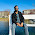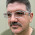## Jan 25, 2012

### DMQL OR DMQL2 Tutorial

DMQL OR DMQL2 (Data mining query language) tutorials for RETS with examples

In RETES to get the data from MLS sever it uses DMQL. DMQL has two parts:

1. Select statement
2. Search condition

Note: In DMQL we can use only system name or standard name of fields. Default is system name. If you want to use standard name then you must have to set StandardNames = 1

Select statement: It is very simple. We have to pass the only field name delimited comma. For example:
Acres,ListPrice,RoomArea,Country,City

Search condition: It is most difficult part of DMQL but we will go through from basic search condition to difficult one. It just like WHERE clause of sql server queries language.

DMQL OR DMQL2 search conditions on numeric fields:

Suppose Price, Price1, Price2 and Price3 are numeric fields of any Resource (You can think it as database or schema) and class (You can think it as table).

1.
Sql server query: WHERE Price = 10

DMQL query: (Price=10)

Verbal meaning:  Filter only those records where Price is equal to 10.

Note: DMQL is space and case insensitive. That is following all search conditions correct and equivalent:
a.   ( Price =  10  )
b. (PRICE = 10)

2.
Sql server query: WHERE Price >= 10

DMQL query: (Price=10+)

Verbal meaning:  Filter only those records where Price is greater than or equal to 10.

3.
Sql server query: WHERE Price <= 10

DMQL query: (Price=10-)

Verbal meaning:  Filter only those records where Price is less than or equal to 10.

4.
Sql server query: WHERE Price BETWEEN 10 AND 20

DMQL query: (Price=10-20)

Verbal meaning:  Filter only those records where Price is greater than or equal to 10 and price is less than equal to 20.

5.
Sql server query: WHERE Price1 = 10 AND Price2 >= 20

DMQL query: (Price1=10),(Price2=20+)
Or DMQL query: (Price1=10) AND (Price2=20+)

Verbal meaning:  Filter only those records where Price1 is equal to 10 and Price2 is greater than or equal to 20

6.
Sql server query: WHERE Price1 = 10 OR Price2 >= 20

DMQL query: (Price1=10)|(Price2=20+)
Or DMQL query: (Price1=10) OR (Price2=20+)

Verbal meaning:  Filter only those records where Price1 is equal to 10 or Price2 is greater than or equal to 20

7.
Sql server query: WHERE Price1 = 10 AND Price2 != 20

DMQL query: (Price1=10),~(Price2=20)
Or DMQL query: (Price1=10) AND NOT (Price2=20)

Verbal meaning:  Filter only those records where Price1 is equal to 10 and Price2 is not equal to 20

8.
Sql server query: WHERE Price1 IS NULL OR Price2 IS NOT NULL

DMQL query: (Price1=.EMPTY.)|~(Price2=.EMPTY.)
Or DMQL query: (Price1=.EMPTY.) OR NOT (Price2=.EMPTY.)

Verbal meaning:  Filter only those records where Price1 is equal null or price2 is not null

9. Sql server query: WHERE (Price1 >= 10) OR (Price2 = 20 AND Price3 != 50) OR ((Price1 IS NULL OR Price2 BETWEEN 50 AND 100) AND (Price3 <= 100))

DMQL query: (Price1=10+) | ((Price2=20), ~(Price3=50)) | (((Price1=.EMPTY.) | (Price2 50-100)) , (Price3=100-))

Or DMQL query: (Price1=10+) OR ((Price2=20) AND  NOT (Price3=50)) OR (((Price1=.EMPTY.) OR (Price2 50-100)) AND (Price3=100-))

DMQL OR DMQL2 Tutorial
DMQL OR DMQL2 search conditions on characters or string fields
DMQL OR DMQL2 search conditions on look up fields

1.Anonymous4/26/2012

i like it.

2.Anonymous1/24/2014

Thanks for providing info on this obscure topic - unified.sng@gmail.com

3.Anonymous9/07/2014

really nice tutorial

4.Anonymous9/12/2015

I'm using DMQL2 and any of the number operators (2-3, 3+ etc.) do not seem to be working anymore. %2B seems to replace + for these operators.

5.I have to retrieve the data from multiple resources. Is there any way to do so.
For example:

I have resources and classes like:

1. Property(Resource), Listing(Class)
2. Media(Resource), Media(Class)
Some data should be fetched from the first and a little bit from the second.

I am using the Phrets to retrieve the data from the Rets server.

6.I am trying to figure out how to pull data back for the last three years
I can do it using L_ClosingDate=2013-10-04+
but how do I get a rolling last three years?
Someone told me L_ClosingDate=(TODAY-3Y)+ but I can't get that to work.

1.Hi Alex,

I know this is an old post, but did you ever get this to work?

7.Anonymous10/05/2016

8.